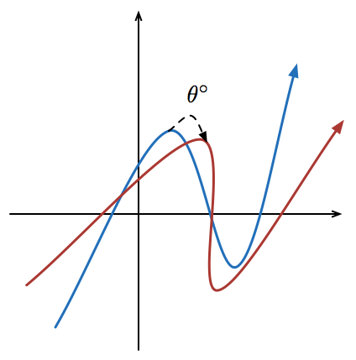# Rotating a Graph?

Calculus Level 5The above shows twos functions on a same Cartesian Plane: $f(x)$ and $f^\theta (x)$ colored blue and red respectively.

Let $f(x)=x^3-6x^2+6x+1$

Define $f^{\theta}(x)$, where $\theta \geq 0$, as the new graph obtained by rotating the graph of $f(x)$ $\theta$ degrees about the origin ($0,0$) in the clockwise direction.

Find the maximum value of $\theta_M$ such that for all values of $0<\theta<\theta_M$, $f^{\theta}(x)$ has every value of $x$ paired with only one $y$ (more simply, no two points can have the same $x$-coordinate).

Input your answer as $\lfloor 100\cdot\theta_m \rfloor$. Where $\lfloor x \rfloor$ is the floor function which means the greatest integer less than or equal to $x$.

This is part of the set Trevor's Ten

×